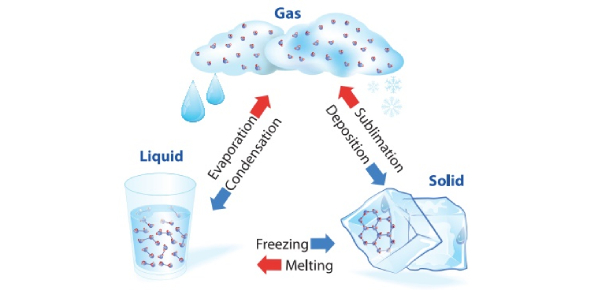# Water: Change Of States Quiz!

10 Questions | Total Attempts: 33Settings.

• 1.
The diagram below shows the change of states of a substance. The different processes are represented by A, B, C, and D. Which one of the following correctly represents A, B, C, and D?
• A.

Freezing, Condensation, Melting, Boiling

• B.

Boiling, Freezing, Condensation, Melting

• C.

Melting, Boiling, Condensation, Freezing

• D.

Condensation, Melting, Boiling, Freezing

• 2.
A kettle containing some tap water was heated until it boiled. White clouds were seen coming out of the spout. A metal tray was placed above the spout of the kettle. Which of the following statement about the experiment is not correct?
• A.

The white clouds were made up of tiny water droplets

• B.

The tap water gained heat and turned into gaseous state which were the white clouds.

• C.

The metal tray became warm eventually and fewer water droplets were formed on the tray

• D.

The hot water vapour from the spout touched the cooler surface of the metal tray, lost heat and condensed into water droplets

• 3.
The table below shows the melting points of substances W, X, Y, and Z. Which of the substance(s) will be in the liquid state at 35oC?
• A.

W only

• B.

W and X only

• C.

X and Z only

• D.

X, Y, and Z only

• 4.
Three glasses A, B, and C were filled with the same amount of water. the temperature of the water is different in each glass. After 15 minutes, water droplets were seen on B and C as shown below. no water droplets were seen on A. Which one of the following correctly shows the arrangement of the glasses based on the temperature of water it holds? (highest water temperature --> lowest water temperature)
• A.

A --> B --> C

• B.

B --> A --> C

• C.

C --> A --> B

• D.

C --> B --> A

• 5.
Four students commented on the boiling and evaporation of water. Which two students are correct?
• A.

Aimah and Cally

• B.

Aimah and Dilsha

• C.

Betty and Cally

• D.

Betty and Dilsha

• 6.
Two metal containers, K and M, each contained 200 cm3  of water. Jim recorded the volumes of water in containers K and M every hour. Which of the following is the correct graph for these results?
• A.

Option 1

• B.

Option 2

• C.

Option 3

• D.

Option 4

• 7.
The diagram below represents the water cycle. At which stage(s) is/are heat gained by water?
• A.

X only

• B.

Y only

• C.

Z only

• D.

W and Y

• 8.
The graph below shows how the temperature of water in a beaker changes when heated over time. Which line in the graph shows the boiling point of water?
• A.

AB

• B.

BC

• C.

CD

• D.

BD

• 9.
Two identical cups, A and B were each filled with the same amount of water. The temperature of water in cups A and B were 40oC and 5oC, respectively. They were left in the science room for forty minutes. Which one of the graphs correctly shows the change of temperature for the two cups of water?
• A.

Option 1

• B.

Option 2

• C.

Option 3

• D.

Option 4

• 10.
Which of the following correctly shows whether heat is gained or lost in each of the three processes?
• A.

Option 1

• B.

Option 2

• C.

Option 3

• D.

Option 4

Related TopicsBack to top